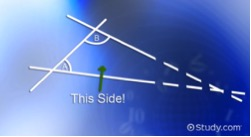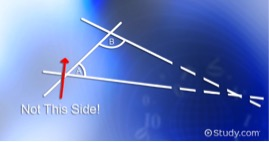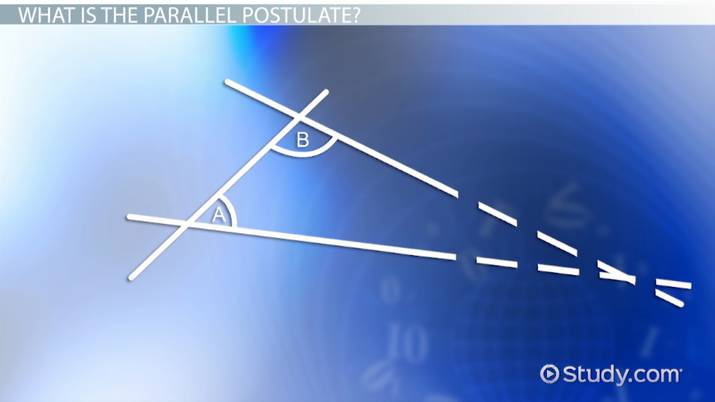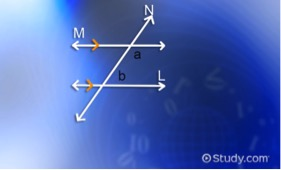# The Parallel Postulate: Definition & Examples

Lesson Transcript
Instructor: Artem Cheprasov

Artem has a doctor of veterinary medicine degree.

In geometry, the parallel postulate argues that if a third line is used to connect two straight lines, and that third line creates two angles on the same side that are each less than 90 degrees, then the two straight lines will eventually intersect. Review examples and recognize why this postulate is important in geometry. Updated: 09/29/2021

## What Is the Parallel Postulate?

Thousands of years ago, a Greek mathematician named Euclid laid out the foundations of plane geometry in five postulates, which are statements assumed to be true without proof. In today's lesson, we will cover his fifth postulate, called the parallel postulate, which states that if a straight line intersects two straight lines forming two interior angles on the same side that add up to less than 180 degrees, then the two lines, if extended indefinitely, will meet on that side on which the angles add up to less than 180 degrees.

Confused? You probably are, and that's okay. Let's simplify what that technical mess of a definition actually means. Suppose we have two lines, as shown here on screen.We draw a third line that intersects the other two and label the interior angles alpha and beta, as shown here.Imagine extending the two lines indefinitely. In our figure, it can be clearly seen that they would eventually intersect.What the parallel postulate claims is that these lines would intersect on the side of the angles alpha and beta only if the sum of these angles is less than 180 degrees. Note that the potential intersection occurs on the side of the angles alpha and beta. That is, we are talking about this side:And not this side:Also note that the postulate states that the intersection would only occur if the sum of alpha and beta is less than 180 degrees. If you're still confused, then why don't we take a closer look at the parallel postulate with some examples? Hands-on stuff never hurts, right?An error occurred trying to load this video.

Try refreshing the page, or contact customer support.

Coming up next: What Are Polygons? - Definition and Examples

### You're on a roll. Keep up the good work!

Replay
Your next lesson will play in 10 seconds
• 0:05 What Is the Parallel…
• 0:48 The Parallel Postulate
• 1:47 The Parallel Postulate-Example
• 3:40 Lesson Summary
Save Save

Want to watch this again later?

Timeline
Autoplay
Autoplay
Speed Speed

## The Parallel Postulate - Examples

We can have three general cases with respect to the parallel postulate, starting out with drawing two parallel lines labeled m and l and a transversal labeled n. We place the angles alpha and beta to the right of the transversal. In the resulting figure, it's easy to see that lines m and l would never cross each other.To unlock this lesson you must be a Study.com Member.

### Register to view this lesson

Are you a student or a teacher?